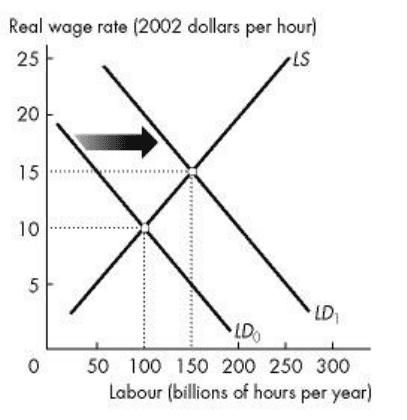Study Guides (390,000)
CA (150,000)
York (10,000)
ECON (900)
ECON 1010 (200)
All (70)
Quiz

# ECON 1010 Study Guide - Quiz Guide: Deficit Spending, Demand Curve, Aggregate SupplyExam

Department
Economics
Course Code
ECON 1010
Professor
All
Study Guide
Quiz

This preview shows pages 1-2. to view the full 8 pages of the document.
Online Quiz 3
3) In 2008, Armenia had real GDP of \$4.21 billion and a population of 2.98 million. In 2009, real GDP
was \$4.59 billion and population was 2.97 million. Between 2008 and 2009, Armenia's standard of living
________.
A) increased
B) decreased
C) did not change
D) might have increased, decreased, or remained unchanged
E) doubled.
8) The Rule of 70 is used to
A) estimate how much of an economy's growth rate is attributable to increases in capital per hour of
labour.
B) calculate the standard of living.
C) calculate the economy's growth rate.
D) estimate how long it will take the level of any variable to double.
E) estimate how much of an economy's growth rate attributable to technological advance is.
14) Suppose a country is producing \$20 million of real GDP. If the economy grows at 10 percent per
year, approximately how many years will to take for real GDP to grow to \$80 million?
A) 14
B) 7
C) 4
D) 30
E) 3.5 years
1) Between 1926 and 2007 real GDP per person in Canada grew at an average rate of
A) 2.8 percent a year.
B) 1.7 percent a year.
C) 2.1 percent a year.
D) 3.6 percent a year.
E) 4.3 percent a year.
7) Between 1926 and 2007, the average growth rate of real GDP per person in Canada was ________
percent a year. During this period, ________ grew at a faster rate than ________.
A) 2.1; GDP; the population
B) 2.1; real GDP; the population
C) 1.1; inflation; real GDP
D) 3.1; the population; real GDP
E) 3.1; inflation; real GDP
4) According to the law of diminishing returns, along the aggregate production function an additional unit
of
A) capital produces more output than an additional unit of labour.

Only pages 1-2 are available for preview. Some parts have been intentionally blurred.B) labour decreases output.
C) labour produces more output than the previous unit.
D) labour produces less output than the previous unit.
E) labour increases the real wage rate.
2) When the quantity of labour demanded exceeds the quantity of labour supplied, the real wage rate
A) rises to eliminate the labour market shortage.
B) falls to eliminate the labour market surplus.
C) rises to eliminate the labour market surplus.
D) falls to eliminate the labour market shortage.
E) does not change but the money wage rate rises to eliminate the labour market shortage.
5) Labour productivity is
A) real GDP per hour of labour times the hours of work.
B) real GDP per hour of labour times the population.
C) the quantity of real GDP produced by an hour of labour.
D) the rate of change in real GDP per hour of labour.
E) none of the above.
6) An increase in population results in
A) an upward shift in the production function.
B) a movement along the production function.
C) a leftward shift of the labour supply curve.
D) a rightward shift of the labour demand curve.
E) both B and D are correct.
Use the figure below to answer the following question.
Figure 22.3.3

Unlock to view full version

Only pages 1-2 are available for preview. Some parts have been intentionally blurred.

25) Refer to Figure 22.3.3. As a result of the rightward shift in the demand curve for labour from LD0 to
LD1, the equilibrium level of employment ________, potential GDP ________, and potential GDP per
hour of labour ________.
A) increases; increases; increases
B) increases; decreases; increases
C) decreases; increases; decreases
D) decreases; decreases; decreases
E) increases; increases; decreases
9) One criticism of the Bank of Canada's focus on an inflation control target is that
A) if inflation falls below the target range a recession will result.
B) if inflation edges above the target range, the Bank decreases aggregate demand and could create a
recession.
C) the Bank pays too much attention to unemployment and real GDP growth and not enough to inflation
control.
D) it makes setting expectations of inflation difficult.
E) the Bank rarely achieves its target.
10) As the sole issuer of Canadian money, the Bank of Canada can set any one of three variables:
A) the monetary base, the exchange rate, and the short term interest rate.
B) the money base, the interest rate, and the unemployment rate.
C) the rate of inflation, the interest rate, and the unemployment rate.
D) the exchange rate, the interest rate, and the inflation rate.
E) the inflation rate, the unemployment rate, and the real economic growth rate.
11) The operating band is
A) the interest rate that is established through an open market operation.
B) the target overnight interest rate plus or minus 25 basis points.
C) the target inflation rate plus or minus a quarter of a percentage point.
D) a half percentage point range in the target inflation rate.
E) the range between the lending rate and the borrowing rate.
16) The purchase of government bonds by the Bank of Canada
A) decreases bank reserves.
B) increases bank loans.
C) decreases the price of bonds.
D) fights inflation.
E) tightens credit conditions.
and its liabilities ________.
A) increase; increase; increase
B) decrease; decrease; increase
C) increase; decrease; increase
D) decrease; decrease; decrease